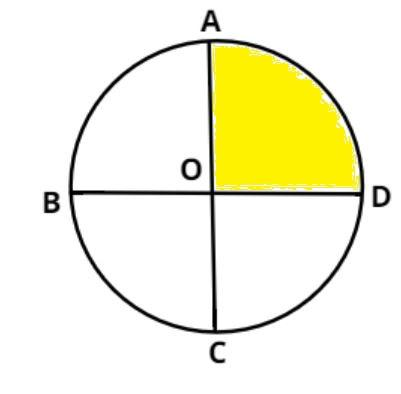Courses
Courses for Kids
Free study material
Free LIVE classes
MoreLIVE
Join Vedantu’s FREE Mastercalss

# Quadrant of a circle is ... ……………………… of the circle.$A.$ One-half.$B.$ One-fourth$C.$ One-third$D.$ Two-thirdsVerified
361.5k+ views
Hint: Divide the circle into four sections by a set of two lines which are perpendicular in nature, so use this concept to reach the solution of the question.Let us consider a circle centered at O.

Let AC and BD are two lines passing through the center and are perpendicular to each other so these two lines divide the circle into four equal portions or we can say in four quadrants.

So, one quadrant is equal to (1/4) of the circle as shown in figure.

So, the quadrant of a circle is one-fourth of the circle.

So, this is the required answer.

Hence option (B) is correct.

Note: In such types of questions first divide the circle into four equal parts as shown in figure it will give us a clear picture of the quadrant so, from the figure we can say that the quadrant of a circle is one-fourth of the circle which is the required answer.
Last updated date: 25th Sep 2023
Total views: 361.5k
Views today: 5.61k